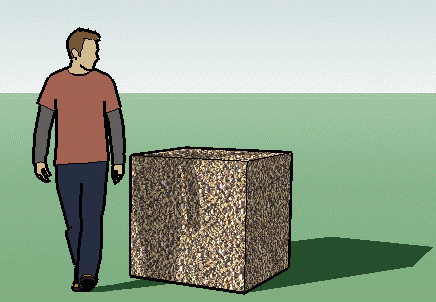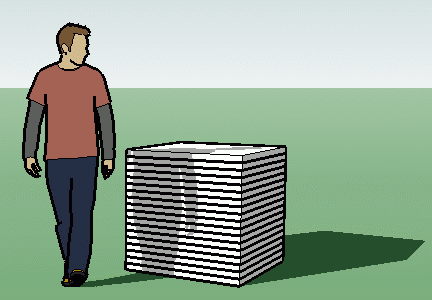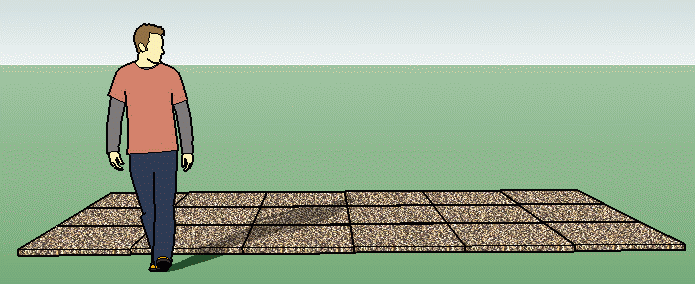SEARCH HOMEMath Central Quandaries & QueriesQuestion from Duncan: I'm trying to figure out how much area 1 cubic yard of gravel would cover at a depth of 2 inches? I've asked around and have many different answers.Hi Duncan.

A cubic yard of gravel is one yard × one yard × one yard, perhaps looking like this:If we slice it into 2 inch slices, we have 36/2 = 18 slices, each of them 2 inches thick, with a top surface that measures one yard × one yard hence with an area of one square yard.

So if we just lay them out, the total area is 18 × 1 yard2 = 18 yards2.Hope this helps,
Stephen.Math Central is supported by the University of Regina and The Pacific Institute for the Mathematical Sciences.